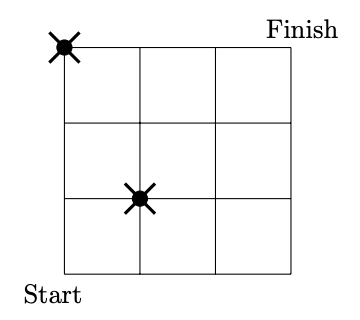시간 제한 메모리 제한 제출 정답 맞은 사람 정답 비율
2 초 64 MB 68 8 5 7.812%

## 문제

Sophie works at a laser factory, which is now introducing a new laser intensifier. This device is a grid, each node of this grid receives and emits photons: for each photon received from the left or down it emits one photon up and one to the right, those photons will be received by nodes in appropriate directions (or are lost if there is no node in this direction). Unfortunately, the industrial scale production is far from being perfect and some of the nodes in the grid are faulty: they neither receive nor emit photons. To be more precise: we know that some nodes are for sure faulty and each other is faulty with the probability $1-p$. Luckily, the exact value of $p$ can be altered by appropriate changes of the pressure during the production. Sophie's task is to compute the value of $p$ such that a single photon emitted to the lower-left node of the grid will, in expectation, yield $k$ photons that are received at the upper-right corner of the grid or establishing that this is not possible. Help her in this task.

Note: if the node in the upper-right corner is faulty then it does not receive any photons.

## 입력

First line of the input contains four positive integers $w$, $h$, $n$, $k$, ($1 \le w, h \le 5\,000$, $0 \le n \le 50$, $1 \le k \le 10^{10000}$), denoting: grids dimensions (width and height), the number of faulty nodes and the number of photons that should be received at the node $(w-1,h-1)$. The following $n$ lines contain the descriptions of faulty nodes, one per each line. In each of those lines there are two positive integers $x, y$ ($0 \le x < w$, $0 \le y < h$); these are the coordinates of the faulty node. All those nodes are pairwise distinct.

## 출력

You should write one real number: the requested probability $p$ . The answer is accepted if such a probability exists and the absolute or relative error is at most  $10^{-6}$. If such a probability does not exist, you should write $-1$. The answer $-1$ is accepted if indeed such a probability does not exist.

## 예제 입력 1

4 4 2 5
0 3
1 1


## 예제 출력 1

0.953069489


## 예제 입력 2

3 4 1 10
0 1


## 예제 출력 2

-1


## 힌트

In Sample 1, the situation looks like on picture below: photon starts in grid node marked Start while photon sensor is in grid node marked Finish.In Sample 2, even for $p = 1$ only $4$ photons reach the upper-right corner.